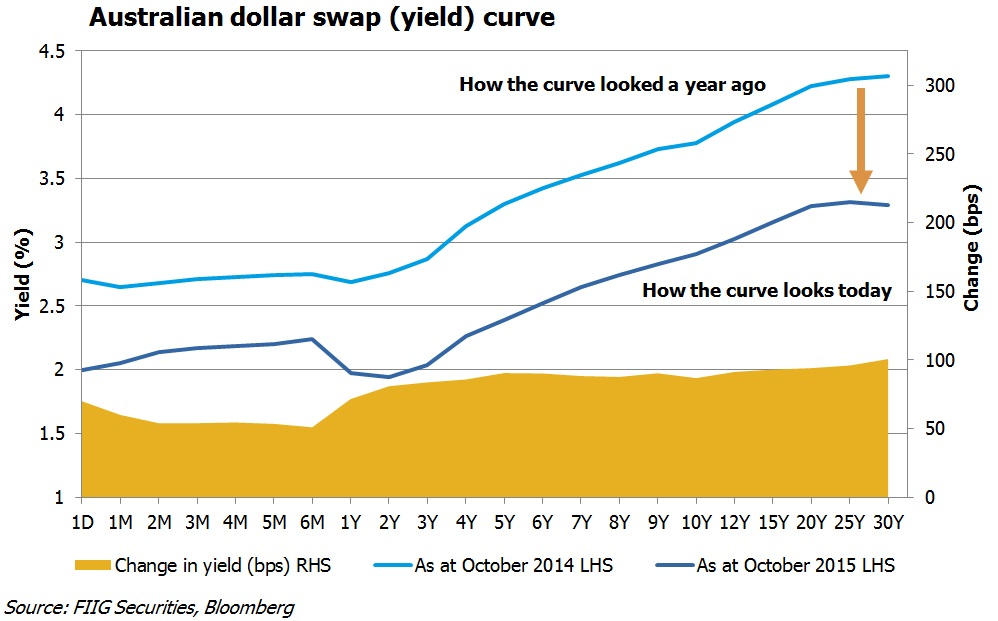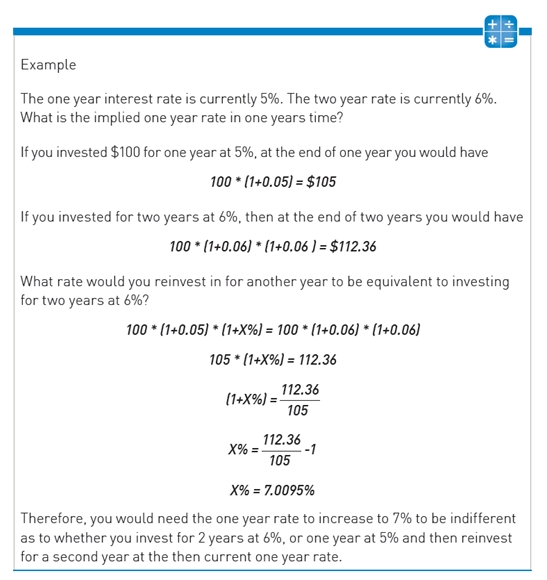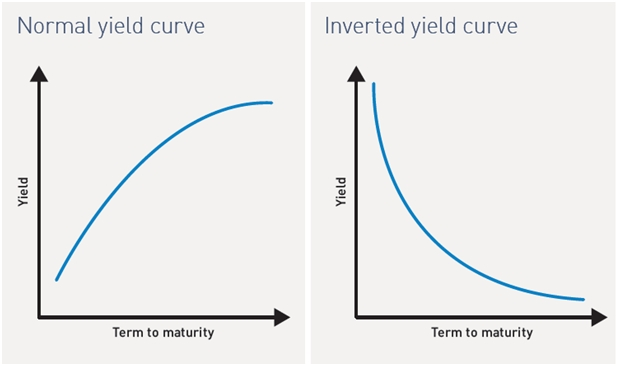Tuesday 06 October 2015 Education (advanced)

# What you need to know about the yield curve

The concept of a yield curve is important as it shows the relationship between interest rates and term to maturity. This note compares the yield curve 12 months ago to the yield curve after the cash rate announcement today. It also provides some background into how it is calculated## The current yield curve

Figure 1 below plots the current Australian dollar swap (yield) curve – the dark blue line. Note that the 2 year maturity point dips below 2.0%, the current RBA cash rate. This shows the market expects rates to be lower than 2.0% on average over the coming two years and hence suggests that rates will fall further before rising over the longer term.Figure 1

The light blue line in Figure 1 above plots where the Australian dollar swap (yield) curve was one year ago and the yellow shading demonstrates the reduction in yields over the year. It is clear to see that compared to one year ago, the market now expects significantly lower yields (around 50 – 100bps lower across the curve) as a result of a number of RBA rate cuts and a less optimistic economic outlook in China and hence Australia.

The current curve and change over the past year are a strong indication of our ‘lower for longer’ view of interest rates. The current curve has the 3 year swap rate at 2.0%, suggesting that the RBA cash rate will average circa 2.0% for the next three years, remembering that is where the cash rate currently resides.

## Yield curve background

The yield curve, also called the term structure of interest rates, shows the relationship between interest rates and term to maturity. Graphically, it plots interest rates on the y-axis with the term to maturity (in years) on the x-axis (as shown in Figure 1). When constructing a yield curve it is important to use interest rates for the same currency and credit quality as these other factors also effect interest rates at different maturities.

The most frequently referenced yield curves are the Commonwealth bond curve which plots yields for risk free Commonwealth government bonds versus maturity and the swap curve which plots yield for current coupon interest rate swaps versus maturity. Given there is not a continuous series of observable bonds or yields for a particular class of securities, the yield curve is constructed by taking market observable yields for discrete maturities and then interpolating between these maturities to create a smooth yield curve. The resulting continuous yield curve can then be used to value cashflows occurring at various future dates, a principal behind the valuation of fixed income securities.

The yield curve is the market’s current view of interest rates for various terms to maturity. However, the yield curve can also be used to determine the market’s current view of future interest rates. For example, by observing the current yields for one year and two year government bonds an investor can determine the market’s current view of the one year government bond rate in a years’ time. Importantly, this is not the actual one year government bond rate in a years’ time but the market’s current forecast of the one year government bond yield in a years’ time. As the current yield curve changes so too does the implied one year government bond yield in a years’ time. By extending this methodology for other maturities an investor can create a yield curve that shows the expected rates for various maturities in a years’ time. This is called the forward curve, or in this case, the one year forward curve. It shows the expected yields for various maturities in one years’ time, based on today’s current yield curve.There are two main patterns created by the term structure of interest rates:

1. A normal yield curve.

2. An inverted yield curve.

## Normal yield curve

In a normal or positive yield curve environment long term fixed income securities offer higher yields than short term fixed income securities. This is intuitive since investors require a premium to compensate for the uncertainties associated with the general economic climate and the financial viability of the issuer over the long term (see Figure 2). Under these conditions, fixed rate bond prices decrease and yields increase. Floating rate notes show greater capital stability as the coupons grow with increasing interest rates.Source: FIIG Securities
Figure 2

## Inverted yield curve

In an inverse or negative yield curve environment the market expects interest rates to decline as time progresses, which is represented by shorter dated yields being higher than longer dated yields (see Figure 2). Remember also, that as interest rates decrease, fixed rate bond prices increase and yields decline. Inverse yield curves usually occur when the central bank is aggressively tightening monetary policy in an attempt to slow the economy and limit inflation. We saw this in Australia around 1990–91 where cash rates rose to 18% while long term bonds yielded 14–15%.

> View all

> View all

## Contributors

• Jonathan Sheridan• Asmita Kulkarni• Thomas Jacquot• Kieran Quaine• Casper Wolski• Jessica Rusit• Trading Desk• Lincoln Tragardh• FIIG Research• Guest Contributor> more